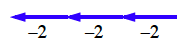### Home > MC1 > Chapter 2 > Lesson 2.2.4 > Problem2-93

2-93.

Find the value of each of the following expressions.

1.  $3(4)$

Reminder: The parentheses used in this problem mean that you should multiply the numbers. If you are having trouble with multiplication, it may help to refer to the definition in your text book on page 101.

1.  $4+11+(−4)$

Remember that adding a negative number is just like taking steps backward, or subtracting.

1.  $3(2)$

Test your multiplication skills. Also, make sure you don't use a calculator!

1.  $−2+(−2)+(−2)$

Again, adding negative numbers is like walking backwards. Does it help to think of the expression as a tightrope?The sum of this expression is $−6$.

1.  $2(−7)$

Just as with addition, when you multiply negative numbers, you take movements backward. This means the product (result of multiplication) will be negative, too.

1.  $10(−5)$

See the hint in part (e).

The product of this expression is $−50$.